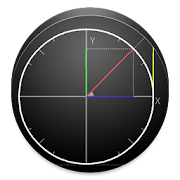# Unit Circle (Trigonometric) - Lumos Educational App Store4.21
Price -fREE
\$0

#### DESCRIPTION:

The unit circle or trigonometric circle is a circle whose center is located at the origin of the Cartesian plane and its radius is 1 (one). It is used in the study of trigonometric functions such as sine, cosine and tangent. - Understand how the projections of the arcs sine, cosine and tangent work in the unit circle (or trigonometric circle); - Why the values ââof sine and cosine are limited to -1 and +1; - Why the tangent of 90 Â° and 270 Â° are not defined - When and where the values ââof the trigonometric ratios (sine, cosine, tangent) are positive, nega

#### OVERVIEW:

Unit Circle (Trigonometric) is a free educational mobile app By .It helps students in grades HS practice the following standards HSF.TF.A.2.

This page not only allows students and teachers download Unit Circle (Trigonometric) but also find engaging Sample Questions, Videos, Pins, Worksheets, Books related to the following topics.

1. HSF.TF.A.2 : Explain how the unit circle in the coordinate plane enables the extension of trigonometric functions to all real numbers, interpreted as radian measures of angles traversed counterclockwise around the unit circle.

HS

#### STANDARDS:

HSF.TF.A.2

Developer:

Software Version: 1.03

Category:

### RELATED APPSEdSearch WebSearch